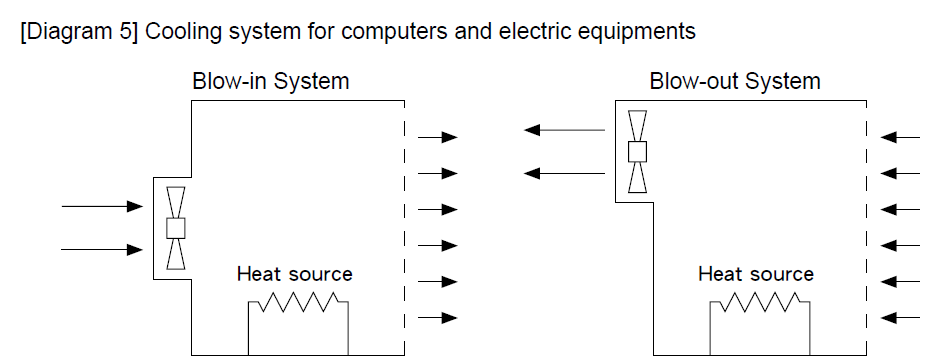# How to select the right GLOBEFAN DC cooling fan, DC blower (part 2)By R&D dept.

Three important steps to select the right fans and blowers are listed below. They help to meet the above-mentioned targets.

Step 1: Define the equipment’s specifications and conditions.
Base on the input and output currents, make sure the temperature rise and the entire heat generated.

Step 2: Calculate the airflow to dissipate the heat. The basic formula for heat transformation of airflow:
H = Ca × Ma × ΔT
H: Transformed heat in watt (W)
Ca: Specific heat of air
Ma: Weight of output air (Q×ρa)
ρa: Air density
ΔT: Temperature rise from system
Q = H/Ca×ρa×ΔT
From which ρa = 3160kW/°F = 1756 kW/°C, then
Q(CFM) = 3.160×P/ΔTF = 1.756×P/ΔTC
Q(CMM) = 0.090×P/ΔTF = 0.050×P/ΔTC
Q: airflow required for cooling
P: power consumption in Watt (W)
ΔTF: temperature allowable in Fahrenheit
ΔTC: temperature allowable in Celsius
ΔT = ΔT1 – ΔT2